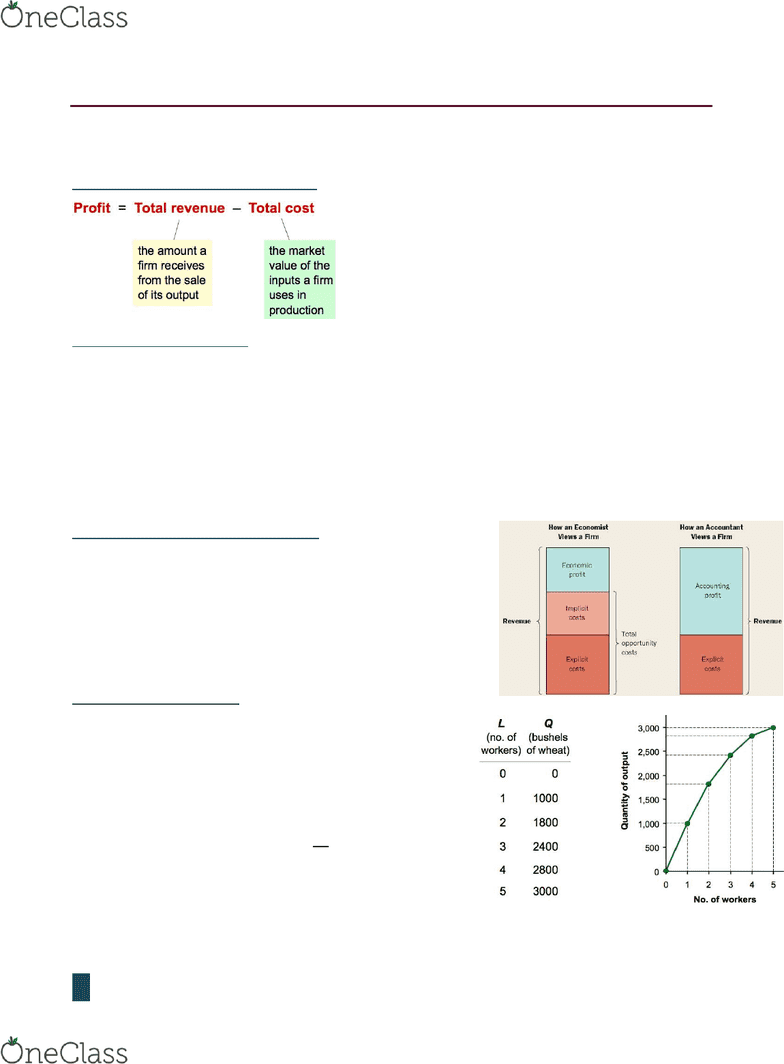Textbook Notes (280,000)
CA (160,000)
Ryerson (10,000)
ECN (800)
ECN 104 (300)
Chapter 13

# ECN 104 Chapter Notes - Chapter 13: Marginal Product, Opportunity Cost, Production Function

Department
Economics
Course Code
ECN 104
Professor
Chapter
13

This preview shows half of the first page. to view the full 3 pages of the document.Parmida
1
Chapter 13 The Cost of Production
WHAT ARE COSTS?
Total Revenue, Total Cost and Profit
Cost as Opportunity Costs
Explicit costs require an outlay of money, e.g. paying wages to workers
Implicit Costs do’t euie a cash outlay, e.g. the oppotuity cost of the oe’s tie
You need \$100,000 to start your business and the interest rate is 5%. Use 40k of your savings,
borrow the other 60k.
- Explicit cost = 3k (5%) interest on loan
- Implicit cost = 2k (5%) foregone interest you could have earned on your 40k
Economic Profit vs Accounting Profit
Accounting Profit = Total revenue Total explicit cost
Economic Profit = Total revenue total costs (explicit +
implicit)
PRODUCTION AND COSTS
The Production Function
- Shows the relationship between the quantity of
inputs used to produce a good and the quantity of
output of that good
- Can be represented by a table, equation or graph
Marginal Product the increase in output arising from an
additional unit of that input   
 = slope of Prod Fun.
Diminishing marginal product the MPL of an input
declines as the quantity increases
find more resources at oneclass.com
find more resources at oneclass.com